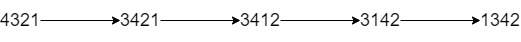# GeetCode Hub

Given a string `num` representing the digits of a very large integer and an integer `k`.

You are allowed to swap any two adjacent digits of the integer at most `k` times.

Return the minimum integer you can obtain also as a string.

Example 1:```Input: num = "4321", k = 4
Output: "1342"
Explanation: The steps to obtain the minimum integer from 4321 with 4 adjacent swaps are shown.
```

Example 2:

```Input: num = "100", k = 1
Output: "010"
Explanation: It's ok for the output to have leading zeros, but the input is guaranteed not to have any leading zeros.
```

Example 3:

```Input: num = "36789", k = 1000
Output: "36789"
Explanation: We can keep the number without any swaps.
```

Example 4:

```Input: num = "22", k = 22
Output: "22"
```

Example 5:

```Input: num = "9438957234785635408", k = 23
Output: "0345989723478563548"
```

Constraints:

• `1 <= num.length <= 30000`
• `num` contains digits only and doesn't have leading zeros.
• `1 <= k <= 10^9`

class Solution { public: string minInteger(string num, int k) { } };